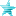# Code optimization (Read 3281 times)

Started by Black_Dahlia_Isis, May 16, 2005, 12:09:10 PM

#### Black_Dahlia_Isis##### Code optimization
#1  May 16, 2005, 12:09:10 PM
••• ~ Esprit guerrier ~
Piece of code with PosAdd, which is a controller that move your char from a specified amount of pixel when triggered :

Code:
`[State 2450, 3]type = PosAddtrigger1 = AnimElem = 1x = -17[State 2450, 4]type = PosAddtrigger1 = AnimElem = 2x = -8[State 2450, 5]type = PosAddtrigger1 = AnimElem = 3x = 23[State 2450, 6]type = PosAddtrigger1 = AnimElem = 4x = 7[State 2450, 7]type = PosAddtrigger1 = AnimElem = 5x = 10`

If you remember that a true trigger = "1", you can merge all these controllers in one :
(which is far shorter isn't it ?)

Code:
`[State 2450, 3]type = PosAddtrigger1 = AnimElem = 1trigger2 = AnimElem = 2trigger3 = AnimElem = 3trigger4 = AnimElem = 4trigger5 = AnimElem = 5x = ((AnimElem = 1) * (-17)) + ((AnimElem = 2) * (-8)) + ((AnimElem = 3) * 23) + ((AnimElem = 4) * 7) + ((AnimElem = 5) * (-10))`

where "x" for trigger1 true = (1 * (-17)) + (0 * (-8)) + (0 * 23) + (0 * 7) + (0 * -10)
= -17 + 0 + 0 + 0 + 0
= -17 of course, which is the x value for trigger1 true.
... same for other triggers.
Last Edit: May 16, 2005, 04:19:45 PM by Isis, Dahlia Noir

#### Messatsu##### Re: Code optimization
#2  May 16, 2005, 03:59:10 PM
••• In after lock
Check my reply on RS for corrections and expansion.Many people risk their lives everyday by having Mugen.

#### Black_Dahlia_Isis##### Re: Code optimization
#3  May 16, 2005, 04:21:13 PM
••• ~ Esprit guerrier ~
I fixed the copy/paste typos I've forgotten here and there.
For expansion, people still need to check RS.